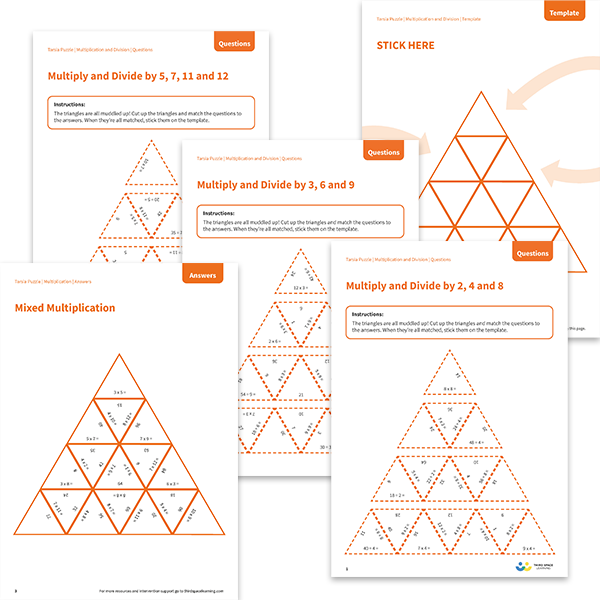# Tarsia Puzzles Mixed Times Tables Pack

The triangles in the tarsia puzzle pack have all been mixed up. In these mixed times table tarsia puzzles, pupils will need to match the multiplication or division question to the correct answer to create one large triangle. These worksheets would be appropriate for Year 4, Year 5 or Year 6.

This mixed times tables pack of tarsia puzzles contains:

• One multiply and divide by 2, 4, and 8 tarsia puzzle (questions, template and answers)
• One multiply and divide by 3, 6 and 9 tarsia puzzle (questions, template and answers)
• One multiply and divide by 5, 7, 11 and 12 tarsia puzzle (questions, template and answers)
• One mixed multiplication tarsia puzzle (questions, template and answers)
• One mixed division tarsia puzzle (questions, template and answers)

Year 4, Year 5, Year 6

Multiplication, Division